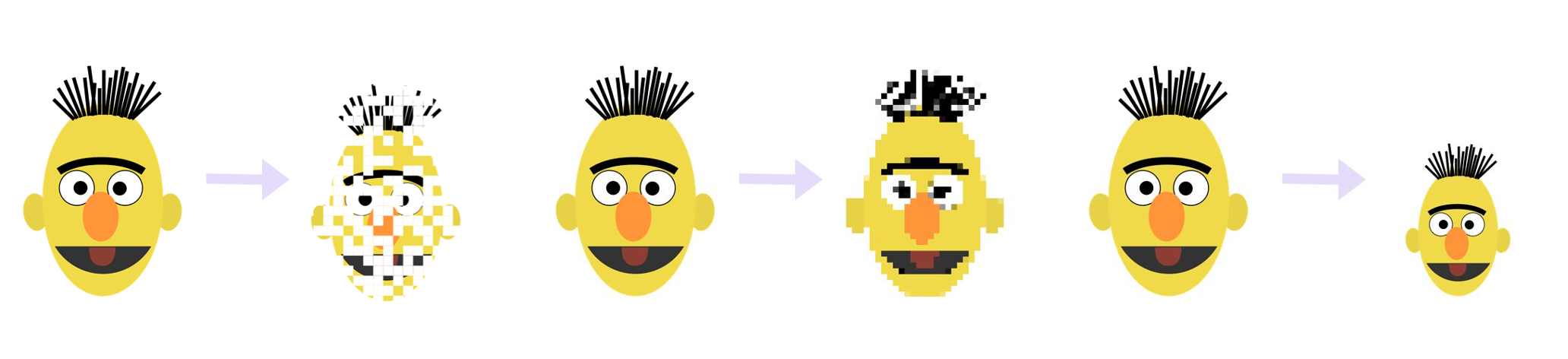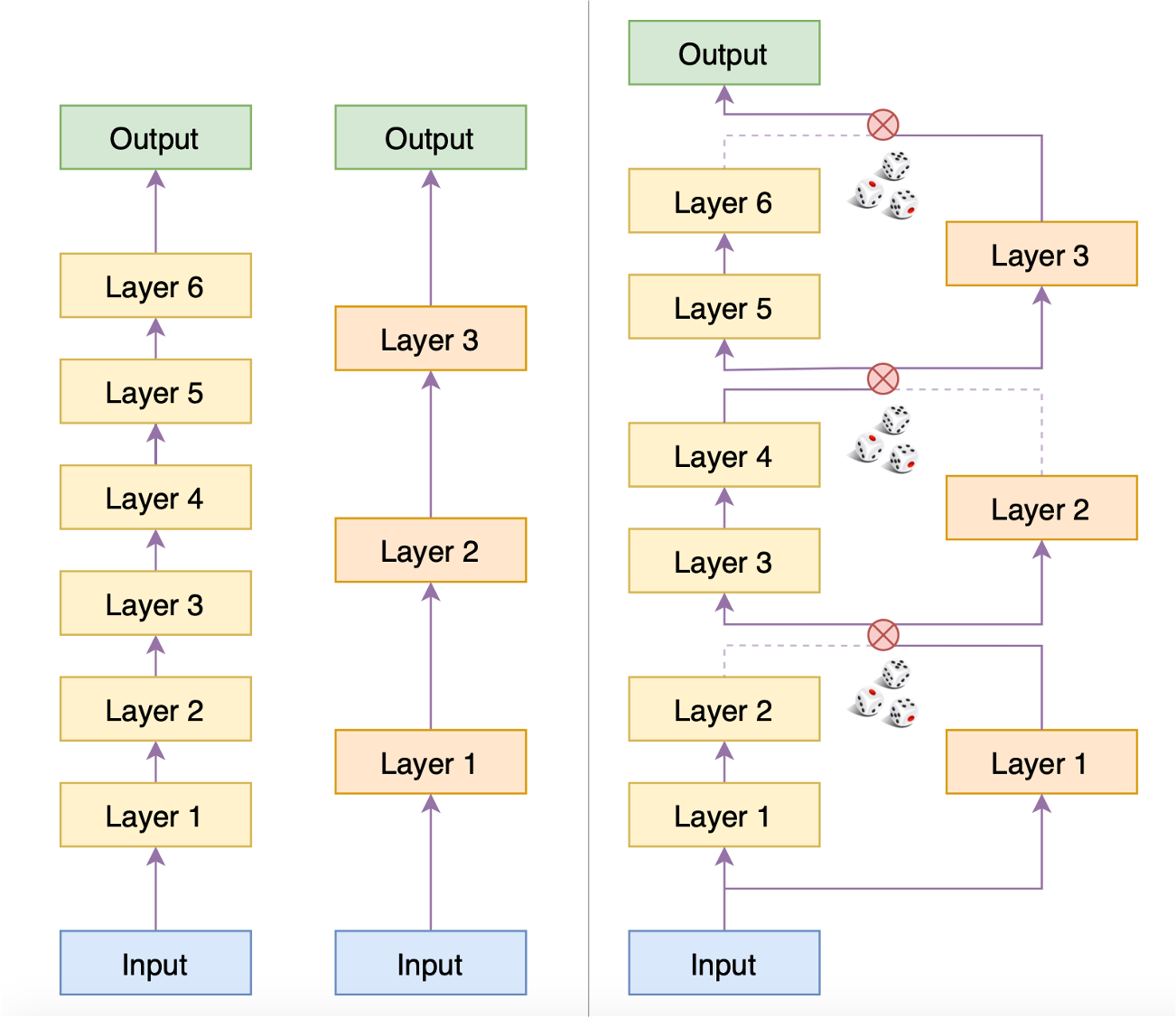# BERT-of-Theseus

## May 29, 2021 • Read: 2763 • Deep Learning • 阅读设置### 模型压缩

#### 常见手段

1. 直接简化大模型得到小模型
2. 借助大模型重新训练小模型### Theseus

BERT-of-Theseus的命名源于思想实验"忒修斯之船"：如果忒修斯船上的木板被逐渐替换，直到所有的模板都不再是原来的木板，那这艘船还是原来的那艘船吗？

#### 流程细节Predecessor和Successor模型示意图（左）
BERT-of-Theseus训练过程示意图（右）

\begin{equation}\begin{aligned} &\varepsilon^{(l)}\sim U(\{0, 1\})\\ &x^{(l)} = x_p^{(l)} \times \varepsilon^{(l)} + x_s^{(l)} \times \left(1 - \varepsilon^{(l)}\right)\\ &x_p^{(l+1)} = F_p^{(l+1)}\left(x^{(l)}\right)\\ &x_s^{(l+1)} = F_s^{(l+1)}\left(x^{(l)}\right) \end{aligned}\tag{1}\end{equation}

#### 方法分析

$\varepsilon$一定要非0即1吗？任意0~1的随机数行不？或者说不随机，直接让$\varepsilon$慢慢地从1变到0行不？这些想法都还没有经过充分实验，有兴趣的读者可以自行实验

#### 实验效果

$$\begin{array}{c|c|c} \hline & \text{直接微调} & \text{BERT-of-Theseus}\\ \hline \begin{array}{c}\text{层数} \\ \text{效果}\end{array} & \begin{array}{ccc}\text{完整12层} & \text{前6层} & \text{前3层} \\ 60.11\% & 58.99\% & 57.96\%\end{array} & \begin{array}{cc}\text{6层} & \text{3层} \\ 59.61\% & 59.36\% \end{array}\\ \hline \end{array}$$